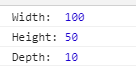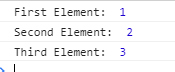# What is the use of curly brackets in the `var { … } = …` statements ?

• Difficulty Level : Medium
• Last Updated : 15 Apr, 2020

Destructuring assignment allows us to assign the properties of an array or object to a bunch of variables that are sometimes very convenient and short. Consider the following illustration. Both of the below-mentioned methods are right and produce the same result.

• Without Destructuring:
```var array = [1, 20, 40];

var first = array
var second = array
var third = arr
```
• With Destructuring
```var array = [1, 20, 40];

var [first, second, third] = array;
```

Object Destructuring Destructuring can be done on JavaScript objects. On the left side of the assignment operator, there is a pattern of variables in which the properties of an object are to be stored. The variable’s name must be the same as defined in the object. Let’s have a look at this concept from the following example.

Note: Curly brackets ‘{ }’ are used to destructure the JavaScript Object properties.

• Example:

 ```<``html` `lang``=``"en"``>`` ` `<``head``>``    ``<``meta` `charset``=``"UTF-8"``>``    ``<``title``>GeeksForGeeks```` ` `<``body``>``    ``<``script``>``     ` `        ``let example_object = {``            ``name: "Object",``            ``platform: "GeeksForGeeks",``            ``number: 100``        ``};``     ` `        ``let {name, platform, number} = example_object;``     ` `        ``console.log("Name: ", name);``        ``console.log("Platform: ", platform);``        ``console.log("Number: ", number);``    `````` ` ``

• Output:If we want the variables defined in the object should be assigned to the variables with other names then we can set it using a colon.

• Syntax:
`{sourceProperty : targetVariable}`
• Example:

 ```<``html` `lang``=``"en"``>`` ` `<``head``>``    ``<``meta` `charset``=``"UTF-8"``>``    ``<``title``>GeeksForGeeks```` ` `<``body``>``    ``<``script``>``        ``let cuboid = {``            ``width: 100,``            ``height: 50,``            ``depth: 10``        ``};``     ` `        ``// width -> w``        ``// height -> h``        ``// depth -> d``        ``let {width:w, height:h, depth:d} = cuboid;``     ` `        ``console.log("Width: ", w);``        ``console.log("Height:", h);``        ``console.log("Depth: ", d);``    `````` ` ``

• Output:Array Destructuring: The elements of the array can also be destructured in the same way. The destructuring assignment can be used to assign the array values to a bunch of different variables in JavaScript.

Note: Square brackets ‘[ ]’ are used to destructure the array elements.

• Example:

 ```<``html` `lang``=``"en"``>`` ` `<``head``>``    ``<``meta` `charset``=``"UTF-8"``>``    ``<``title``>GeeksForGeeks```` ` `<``body``>``    ``<``script``>``        ``var arr = [1, 2, 3];``     ` `        ``var [arr_1, arr_2, arr_3] = arr;``     ` `        ``console.log("First Element: ", arr_1);``        ``console.log("Second Element: ", arr_2);``        ``console.log("Third Element: ", arr_3);``    `````` ` ``

• Output:My Personal Notes arrow_drop_up